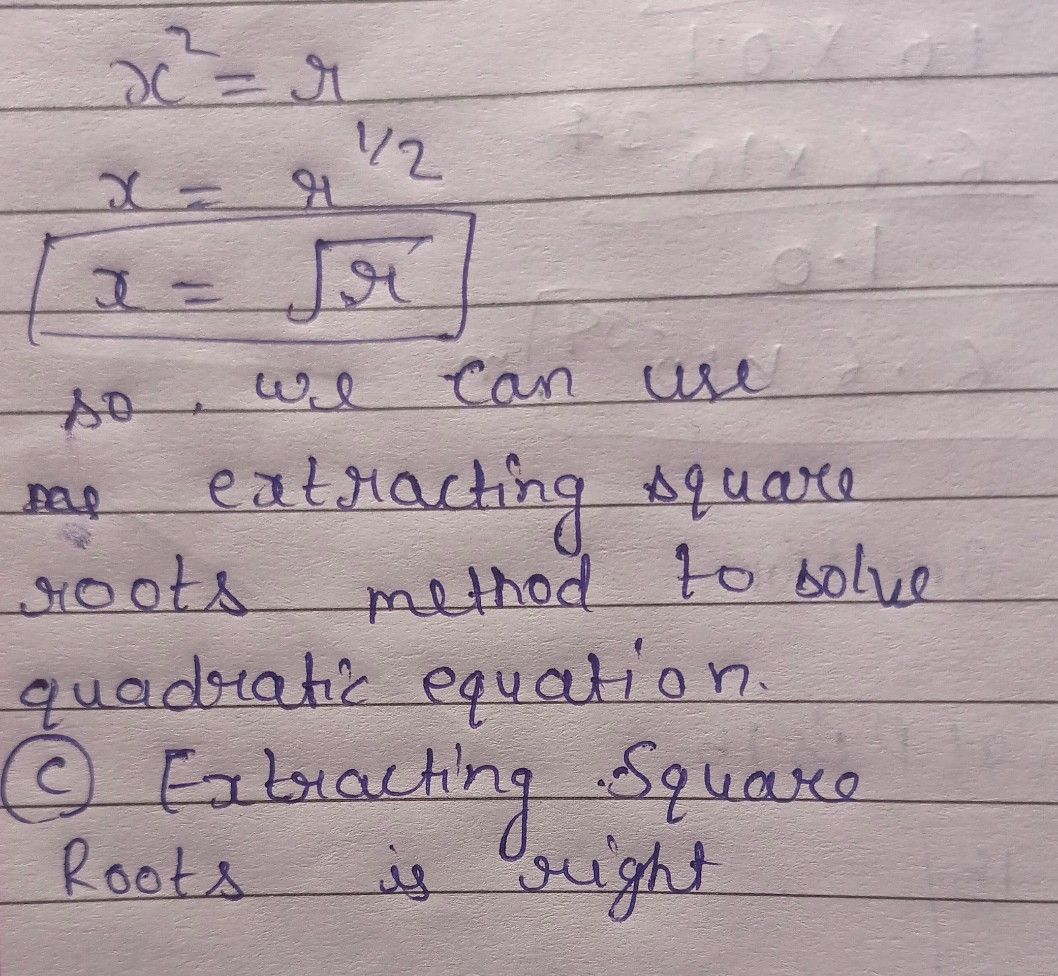Symbol
Problem$1.1$ What method can we use to solve a quadratic equation that can be written in the $1$ form $x^{2}=r7$ A. Quadratic Formula C. Extracting Square Roots $B$ Factoring D. Completing the Square
7th-9th grade
Other
Search count: 107
SolutionQanda teacher - Anshulplease give me review by 5 ?